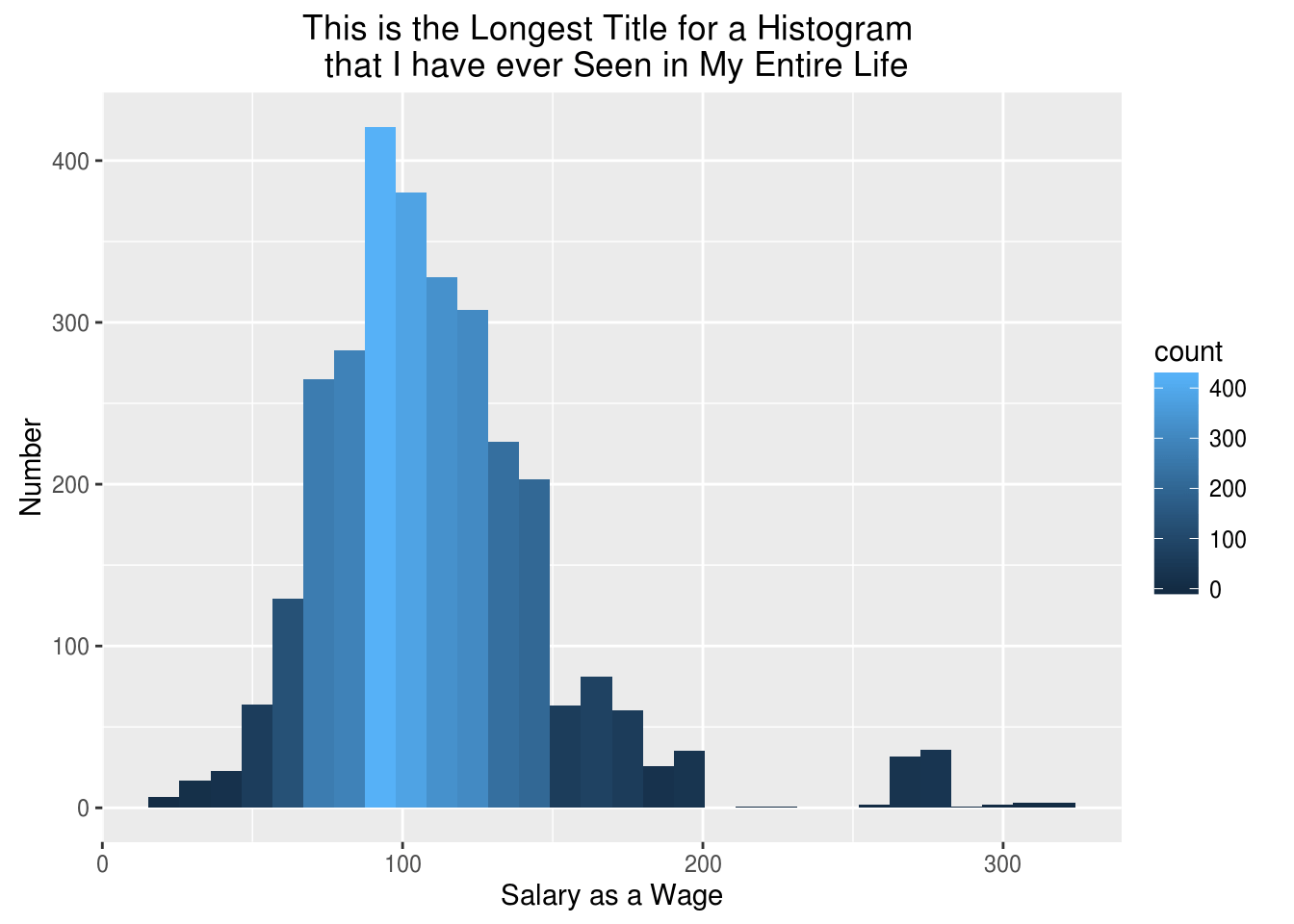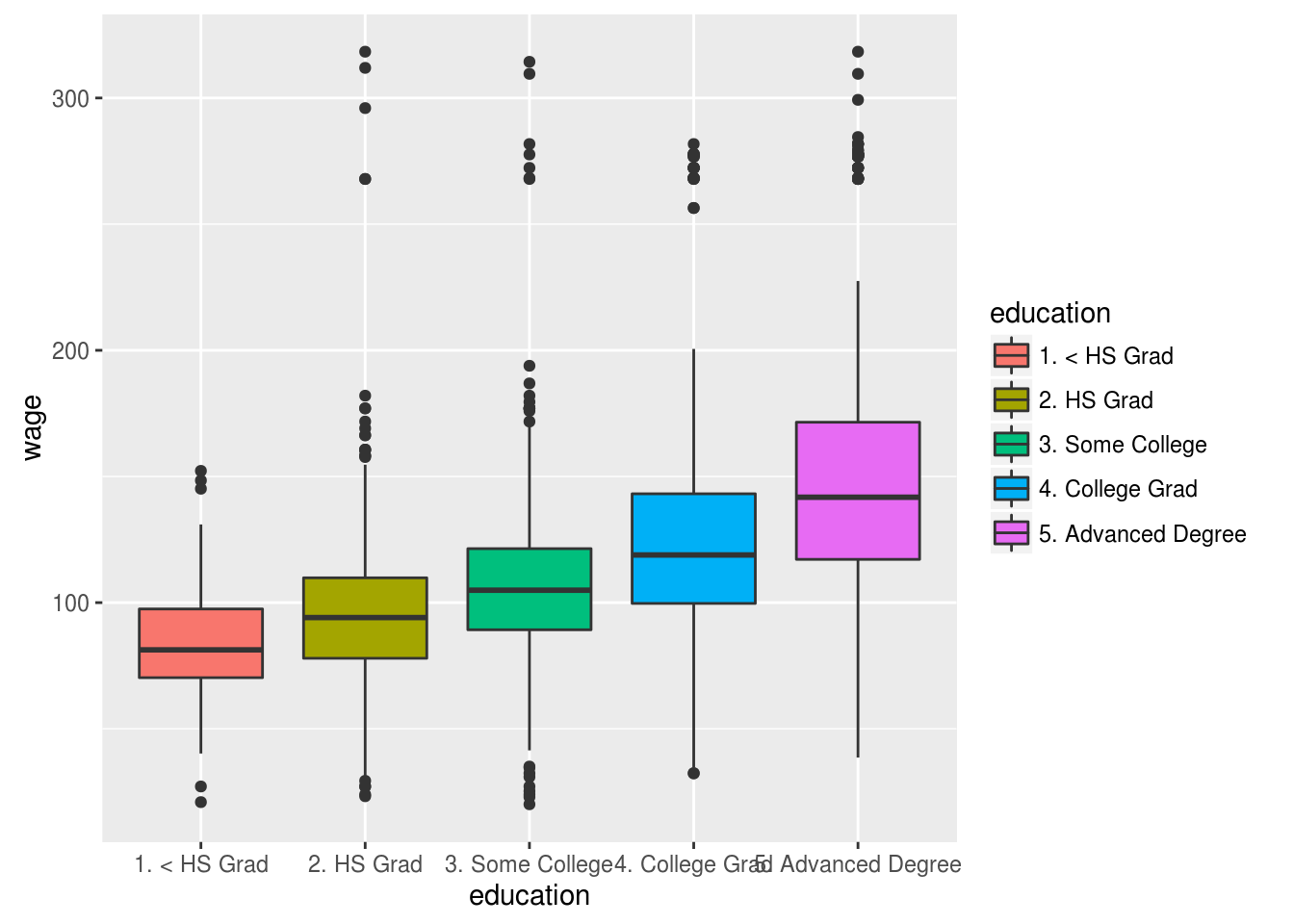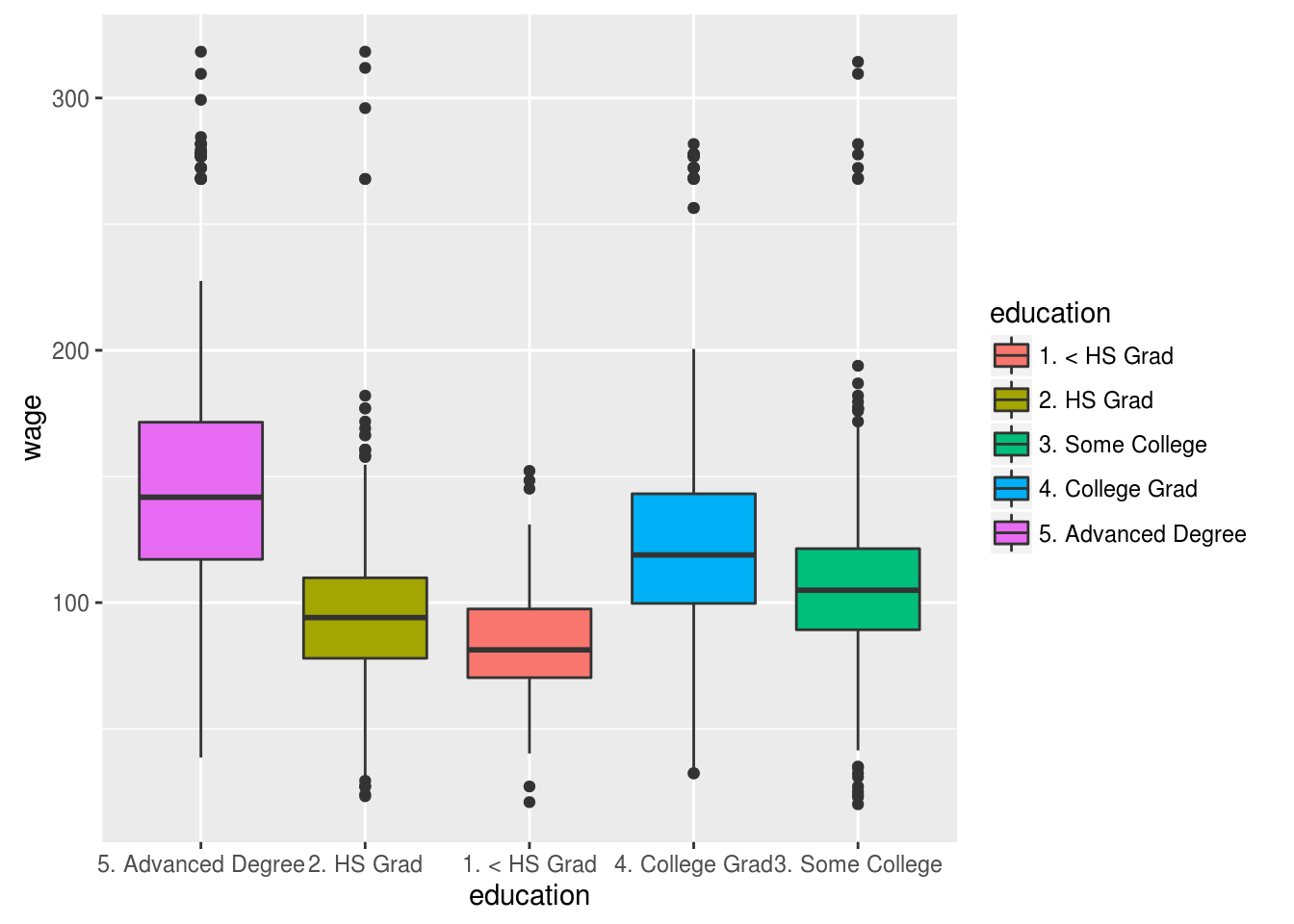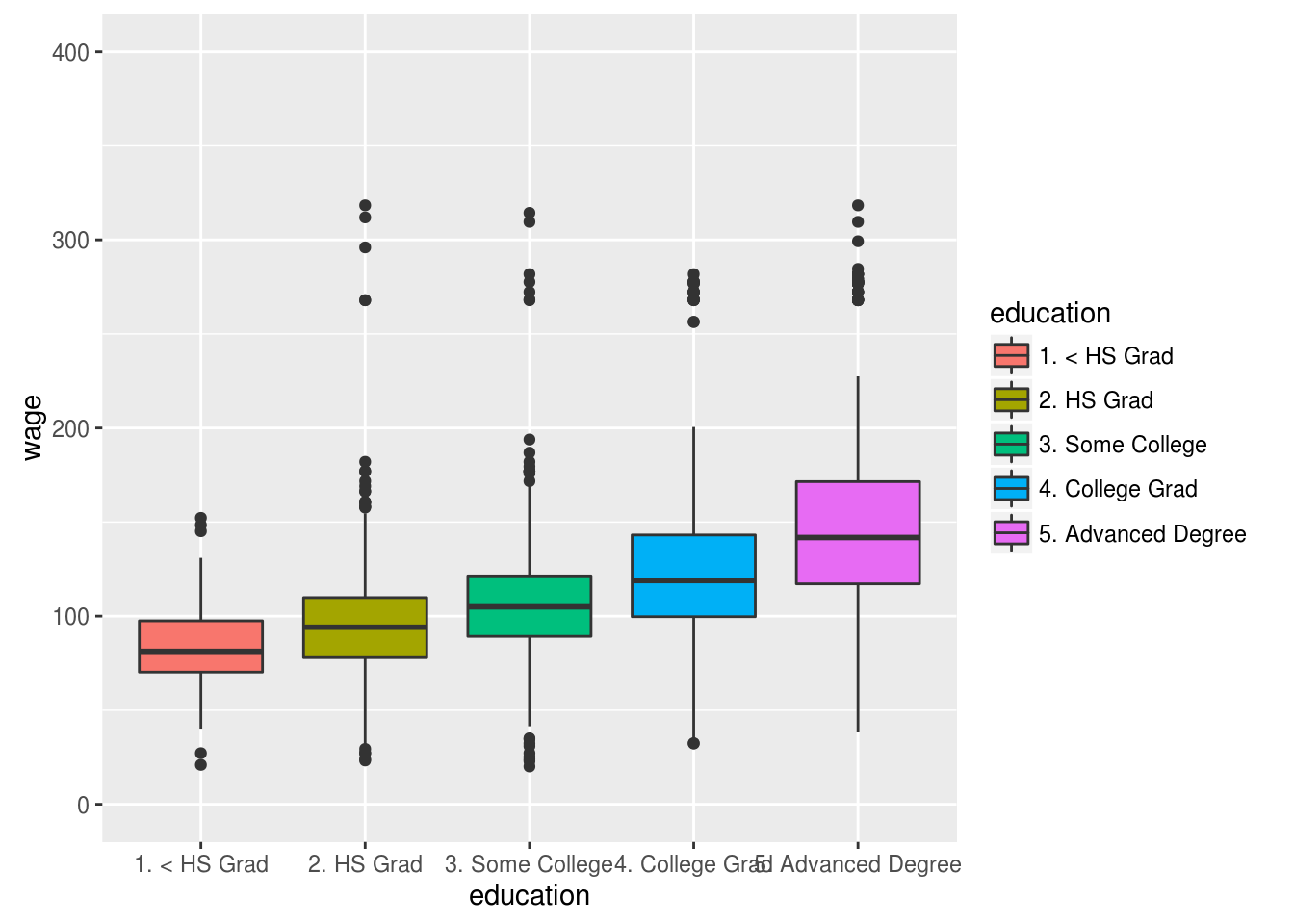# Axis and Labels in ggplot2

In this post, we will look at how to manipulate the labels and positioning of the data when using ggplot2. We will use the “Wage” data from the “ISLR” package. Below is initial code needed to begin.

``````library(ggplot2);library(ISLR)
data("Wage")``````

Manipulating LabelsOur first example involves adding labels for the x, y-axis as well as a title. To do this we will create a histogram of the wage variable and save it as a variable in R. By saving the histogram as a variable it saves time as we do not have to recreate all of the code but only add the additional information. After creating the histogram and saving it to a variable we will add the code for creating the labels. Below is the code

``````myHistogram<-ggplot(Wage, aes(wage, fill=..count..))+geom_histogram()
myHistogram+labs(title="This is My Histogram", x="Salary as a Wage", y="Number")``````
``

By using the “labs” function you can add a title and information for the x and y-axis. If your title is really long you can use the code “” to break the information into separate lines as shown below.

``myHistogram+labs(title="This is the Longest Title for a Histogram \n that I have ever Seen in My Entire Life", x="Salary as a Wage", y="Number")``
``

Discrete Axis Scale

We will now turn our attention to working with discrete scales. Discrete scales deal with categorical data such as box plots and bar charts. First, we will store a boxplot of the wages subsetted by the level of education in a variable and we will display it.

``````myBoxplot<-ggplot(Wage, aes(education, wage,fill=education))+geom_boxplot()
myBoxplot``````Now, by using the “scale_x_discrete” function along with the “limits” argument we are able to change the order of the groups as shown below

``myBoxplot+scale_x_discrete(limits=c("5. Advanced Degree","2. HS Grad","1. < HS Grad","4. College Grad","3. Some College"))``Continuous Scale

The most common modification to a continuous scale is to modify the range. In the code below, we change the default range of “myBoxplot” to something that is larger.

``myBoxplot+scale_y_continuous(limits=c(0,400))``Conclusion

This post provided some basic insights into modifying plots using ggplot2.